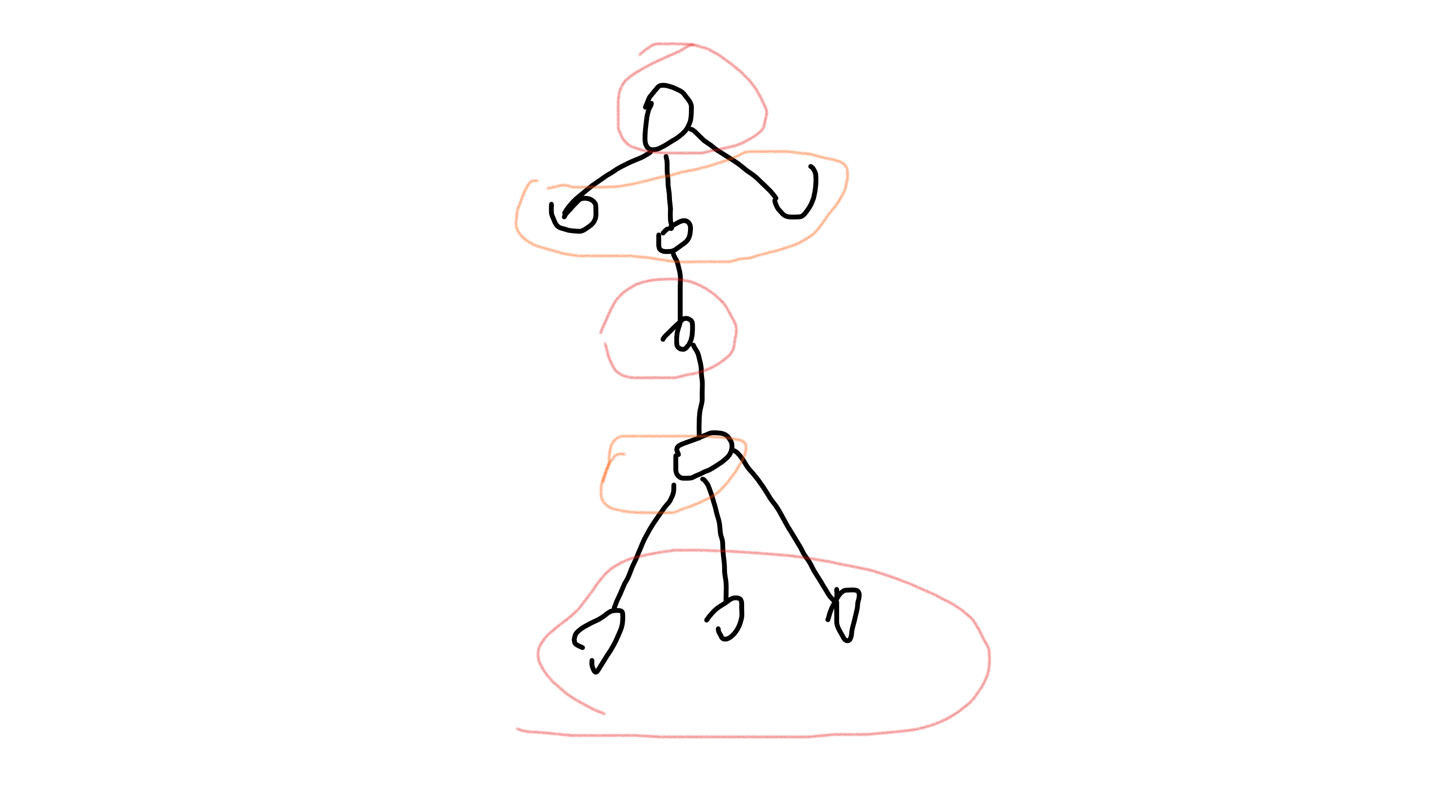# CF1043F - Make It One

（$cnt_i$ 表示以 $i$ 为因数的数的个数，$f_i$ 表示选 $ans$ 个数使得公因数为 $i$ 的方案数。）

（好懒不想写博客）

# 洛谷4767 - [IOI2000]邮局

### （一）

$$f[i][j] = min(f[i][j], f[k][j - 1] + dis(k + 1, i))$$

### （三）

$$f[a][c]+f[b][d]<=f[b][c]+f[a][d]$$

# UVA1220 - Party at Hali-Bula

• $f[u] = \sum\limits_{v \in son[u]} \max(f[v], f[v])$
• $f[u] = \sum\limits_{v \in son[u]} f[v]$

• 如果当前节点 $u$ 更新状态选中的来自 $v$ 的某个状态存在多种，那么 $u$ 的这个状态也存在多种。
• 如果 $u$ 节点的某个孩子 $v$ 满足 $f[v] == f[v]$ ，那么 $f[u]$ 存在多种。

# 洛谷2996 - 拜访奶牛• 如果当前节点不选，那么孩子节点选或不选均可
$f[u] += max(f[G[u][i]], f[G[u][i]])$

• 如果当前节点选中，那么孩子节点只能不选
$f[u] += f[G[u][i]]$